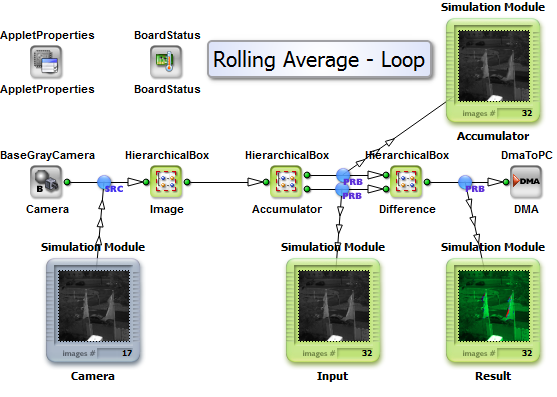### A rolling average is applied on a dynamic number of images

Brief Description

File: \examples\Processing\Loop\RollingAverage\rollingAverage_Loop.vaDefault Platform: mE5-MA-VCL

Short Description

Implementation of a rolling average on a running image stream without introducing a frame delay. The average value of the last N monochrome images is calculated and used to visualize the resulting difference to the current one in color. The number of images being used for building the average value is set to 128, but can be modified.

This design will show how a rolling average will work. But the central element is a loop that will enable much more applications and machine vision operations. In order to understand how a loop works and how it can become implemented, this example is a perfect starting point.

#### Algorithm

A mean value for each single pixel is calculated and normalized to the incoming value range of 8 bit. For this each received frame is summed up with its predecessors and divided by the number of received frames. If the length of the rolling average sequence is reached the sum is reduced by the stored and delayed frame. By this an output image is already generated starting from the first image.

In the design two conditions define the length of the rolling average sequence. Both values are called N_rolling and need to be set to the same value. One is handling the delay store process of substraction images, the other the pixel value normalization.

#### Used Loop

The operators RxImageLink and TxImageLink are used to enable a loop handling. The not normalized average sum of the incoming image sequence is handled in one loop. A second loop handling is required for storing the incoming frames for the later substraction of it values. Each loop needs to be synchronous to the incoming camera frames. This induces a SYNC operator where a start condition needs to be met. The start condition itself is handled by InsertImage where the first run introduces a single minimum frame. The used SYNC maximizes the frame dimensions to the needed value.

Since the average handling needs a certain number of frames being stored the ImageBufferMultiRoiDyn operator is used. The first images are simply stored beside the synchronization purposes are met by minimum frames being generated.

Using the VA simulation will help to understand how this works around SYNC in detail. In general a sequence of artificial minimum frames do the synchronization.

#### Visualization

Two different ways of visualization are implemented. The first will generate a 24 bit RGB image where :

• Plane - Content
• RED - current image
• GREEN - average image
• BLUE - difference image

The second approach is based on a HSL color space that in later converted into 24bit RGB :

• Plane - Content
• HUE / color angle - difference image, where minimum is green
• SATURATION - set to maximum
• LIGHTNESS - current image

The second approach is switched on by EffectEnable = 1. A dynamic gain factor can be used for difference scaling to all Hue color angles.

#### VisualApplets Design

The example "rollingAverage_Loop.va" is designed for a monochrome CameraLink camera in base configuration with a resolution of 1024x1024 at 8bit per pixel.

#### Simulation Data

The example "rollingAverage_Loop.va" folder %VASINSTALLDIR%\examples\Processing\Advanced\RollingAverage\street_sequence*.jpg includes a useful series of images for simulation. A lossy compression format was used in order to reduce amount of data.